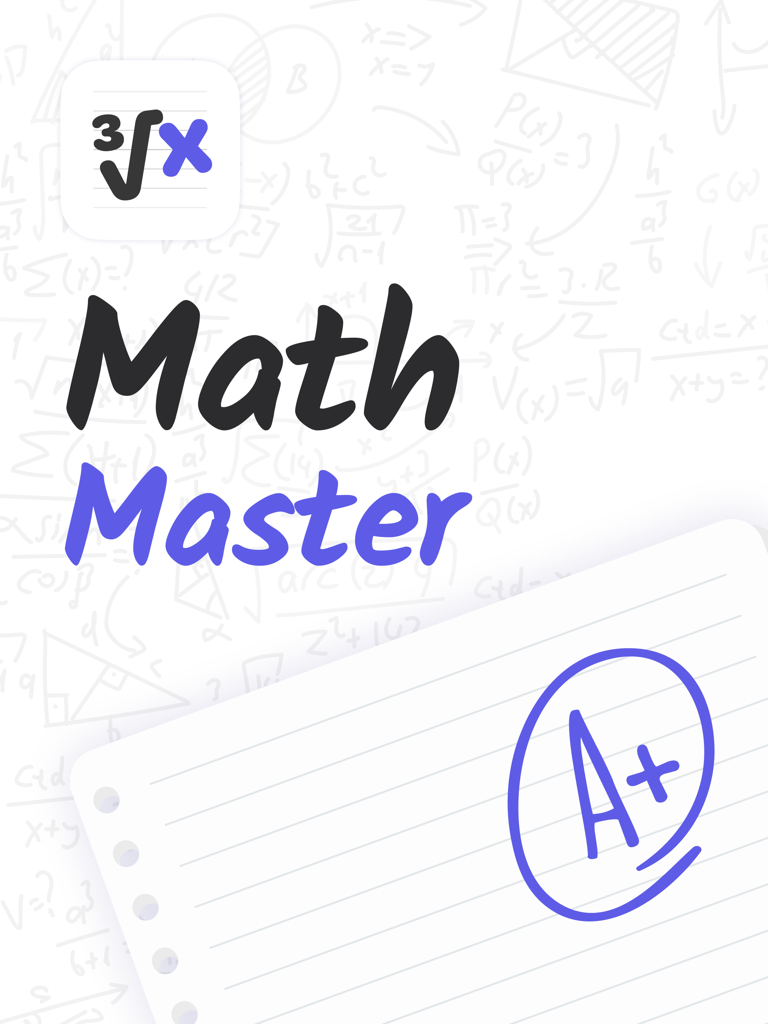# MathMaster: Camera Calculator – Appslux Media LimitedMathMaster: Camera Calculator Appslux Media Limited Genre: Education Release Date: December 31, 2020

MathMaster helps to do the math for you. Just use your camera, snap a picture of a math problem or load an image, and get the right answer on your screen. If you need to get a better understanding of how to solve a problem – just check a step-by-step solution.

The app helps and teaches you how to do your maths homework fast and correctly. All you have to do is to take a photo of the math problem or fill the equation in and press the Enter button.

MathMaster will be your mathematical lifesaver – prepare better for tests, finish your homework faster, and make getting high grades easier. MathMaster also fits for you if you are not strong enough in math solving or need some extra help with it sometimes.

MathMaster covers all levels of math:
• Basic Math/Pre-Algebra: arithmetic, integers, fractions, decimal numbers, powers, roots, factors
• Algebra: linear equations/inequalities, quadratic equations, systems of equations, logarithms, functions, matrices, graphing, polynomials
• Trigonometry/Precalculus: identities, sequences and series, logarithmic functions
• Calculus: limits, derivatives, integrals
• Statistics: combinations, factorials

Printed and Handwritten math problem
MathMaster recognizes both handwritten problems as well as printed ones. Just snap a problem or upload one from your photo gallery.

Step-by-step solutions
MathMaster provides both straight answers and the corresponding step-by-step solutions.

Review an old problem
Go back to old solving anytime. MathMaster will save your results in the "History" tab.

Level up your learning by understanding “how” and “why” math problems are solved, or remember forgotten terms and concepts. Solve math problems instantly across a wide range of topics, ranging from Arithmetic to Calculus.
Math is hard – but with MathMaster, it's never been easier.